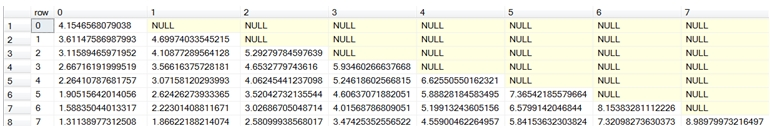# SQL CLR stored procedure for binomial tree

sp_BinomialTree

Updated: 04 Sep 2012

Use the stored procedure sp_BinomialTree to generate a result set of all the values in the binomial tree used to calculate the theoretical price of an option. This stored procedure calls the table-valued function BinomialTree and formats the output into a matrix.
Syntax
DECLARE @CallPut nvarchar(4000)
DECLARE @AssetPrice float
DECLARE @StrikePrice float
DECLARE @TimeToMaturity float
DECLARE @RiskFreeRate float
DECLARE @DividendRate float
DECLARE @Volatility float
DECLARE @nSteps int
DECLARE @AmEur nvarchar(4000)

-- TODO: Set parameter values here.

EXECUTE [wctOptions].[wct].[sp_BinomialTree]
@CallPut
,@AssetPrice
,@StrikePrice
,@TimeToMaturity
,@RiskFreeRate
,@DividendRate
,@Volatility
,@nSteps
,@AmEur
GO
Arguments
@CallPut
identifies the option as being a call ('C') or a put ('P'). @CallPut is an expression of type nvarchar or of a type that can be implicitly converted to nvarchar.
@AssetPrice
the price of the underlying asset. @AssetPrice is an expression of type float or of a type that can be implicitly converted to float.
@StrikePrice
the exercise price of the option. @StrikePrice is an expression of type float or of a type that can be implicitly converted to float.
@TimeToMaturity
the time to expiration of the option, expressed in years. @TimeToMaturity is an expression of type float or of a type that can be implicitly converted to float.
@RiskFreeRate
the annualized, continuously compounded risk-free rate of return over the life of the option. @RiskFreeRate is an expression of type float or of a type that can be implicitly converted to float.
@DividendRate
the annualized, continuously compounded dividend rate over the life of the option. For currency options, @DividendRate should be the foreign risk-free interest rate. @DividendRate is an expression of type float or of a type that can be implicitly converted to float.
@Volatility
the volatility of the relative price change of the underlying asset. @Volatility is an expression of type float or of a type that can be implicitly converted to float.
@nSteps
the number of steps in the binomial tree. @nSteps is an expression of type int or of a type that can be implicitly converted to int.
@AmEur
identifies the option as being American ('A') or European ('E'). @AmEur is an expression of type nvarchar or of a type that can be implicitly converted to nvarchar.
Return Code Values
0 (success) or 100 (failure)
Remarks
·         @Volatility must be greater than zero (@Volatility > 0).
·         @TimeToMaturity must be greater than zero (@TimeToMaturity > 0).
·         @AssetPrice must be greater than zero (@AssetPrice > 0).
·         @StrikePrice must be greater than zero (@StrikePrice > 0).
·         If @ReturnValue is NULL, then @ReturnValue is set to 'P'.
·         If @DividendRate is NULL an error will be returned.
·         If @RiskFreeRate is NULL an error will be returned.
·         @RowNumSteps must be greater than zero.
·         @ColNumSteps must be greater than zero.
Examples
Calculate the price for an American call option on 2012-09-04, expiring on 2012-12-15, with a current asset price of 99.5, a strike price of 100 and a volatility of 20%. The risk free rate is 2% and the dividend rate is 0.5%. The number of steps is 100.
DECLARE @CallPut nvarchar(4000) = 'C'
DECLARE @AssetP float = 99.5
DECLARE @Strike float = 100
DECLARE @Time float = datediff(d,'2012-09-04','2012-12-15')/cast(365 as float)
DECLARE @RiskFree float = .02
DECLARE @Div float = .005
DECLARE @Vol float = .20
DECLARE @nSteps int = 100
DECLARE @AmEur nvarchar(4000) = 'E'

EXECUTE wct.sp_BinomialTree
@CallPut
,@AssetP
,@Strike
,@Time
,@RiskFree
,@Div
,@Vol
,@nSteps
,@AmEur
GO
Here are the first few rows returned by the function.The value returned when row = 0 and col = 0 is the price of the option. The result set is essentially a lower triangular matrix, so the column number will never be greater than the row number.
Since the purpose of the function is to show all the nodes in calculating the theoretical value of the option using the binomial tree method, it can return quite a number of rows and columns.
Remember, that this is a stored procedure and in T-SQL the inputs to the stored procedure are not evaluated. So, if we had used the following syntax,
EXECUTE wct.sp_BinomialTree
'C'
,99.5
,100
,datediff(d,'2012-09-04','2012-12-15')/cast(365 as float)
,.02
,.005
,.20
,100
,'E'
SQL Server will generate an error message. We could, however, have entered
EXECUTE wct.sp_BinomialTree
'C'
,99.5
,100
,0.279452054794521
,.02
,.005
,.20
,100
,'E'
GO
and the stored procedure would have executed and returned the results as above.

### SupportCopyright 2008-2023 Westclintech LLC         Privacy Policy        Terms of Service